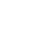Live chatQuestion

Suppose you are a painter, and the price of a gallon of paint increases from \$3.00 a gallon to \$3.50 a gallon. Your usage of paint drops from 35 gallons a month to 20 gallons a month. Perform the following:

Compute the price elasticity of demand for paint and show your calculations.

Ed = (%u2206Qd/QdAverage) ÷ (%u2206P/PAverage)

Where:

Ed represents the price of demand

%u2206Qrepresents the absolute change in quantity

QdAverage  represents the average change in quantity

%u2206P represents the absolute change in price

PAverage represents the average change in price (Arnold, 2008)

 Quantity Demanded Price 35 gallons \$ 3.00 per gallon 20 gallons \$ 3.50 per gallon 15 gallons (change in quantity) \$-0.5 per gallon (change in price)

Incorporating the figures in the formula:

Ed =  {(35-20) ÷ (35+20)/2}÷ {(3.00-3.50) ÷ (3.00+3.50)/2}

Computing the figures represented in the formula:

Ed = {15 ÷ 27.5} ÷ {0.50 ÷ 3.25}

N.B: Note that the result of the change in price was negative but the absolute value is ordinarily positive.

Ed = 0.5454545455 ÷ 0.1538461538

Ed = 3.55

Decide whether the demand for paint is elastic, unitary elastic, or inelastic.

Stay ConnectedIn order to determine whether the demand for the paint is elastic, unitary, or inelastic it will be necessary to determine where the point of elasticity lies when plotted along a straight line demand curve. This is arbitrarily shown below:

Straight Line Demand Curve showing the relative points of occurrence of elasticity of demand.

(Bernanke, 2003)

The price of elasticity of demand is inelastic when it is greater than 1 (Ed>1), while it is unitary when it is equal 1 (Ed=1), and elastic when it is less than 1 (Ed<1) conclusively (Herbert, 2006).

From the figure is clear that the price of elasticity of demand of paint in this scenario is elastic.

The elasticity of demand is considered as being elastic because when plotted on the demand curve, the point falls relatively above the midpoint. According to Webster (2003), “ For all points above the midpoint, the price of elasticity of demand is greater that unity in absolute value…in these cases, demand is said to be elastic” (p.157). In addition, the slope of demand curve primarily determines whether the price elasticity of demand will be classified as elastic or inelastic. Ordinarily, the slope of demand curve usually curves between points A and B, which are expressed in ratio form as represented by the formula and it represents a change in the variables on the vertical axis, which have an effect of variables in the horizontal axis (Arnold, 2008). Moreover, looking at the numerator value or the percentage change in the quantity demanded, if it is greater than its denominator or percentage change in price, the coefficient of elasticity becomes greater than 1 making the demand elastic. Arnold adds that “This is elastic demand; that is, quantity demanded changes proportionately more than the price changes” (p.117).

Limited time Offer

0
0
days
:
0
0
hours
:
0
0
minutes
:
0
0
seconds
Get 19% OFF

The implication therefore implies that a slight change in the price of a particular paint product desired in the market, leads to a significant reduction in the number of buyers of the product. This is especially because there are market forces impacting upon the performance of the product with regard to individual preferences. Some of the market forces include the availability of cheaper alternatives and utility, which impacts upon normal competition variables, consequently affecting the performance of product sales when the price changes (Herbert, 2004). Therefore, increasing prices in the market  has an impact on product performance depending on the acting market forces.

#### Related Economics essays

0

Preparing Orders

0

Active Writers

0

Support Agents

Limited offerget 15% off your 1st order